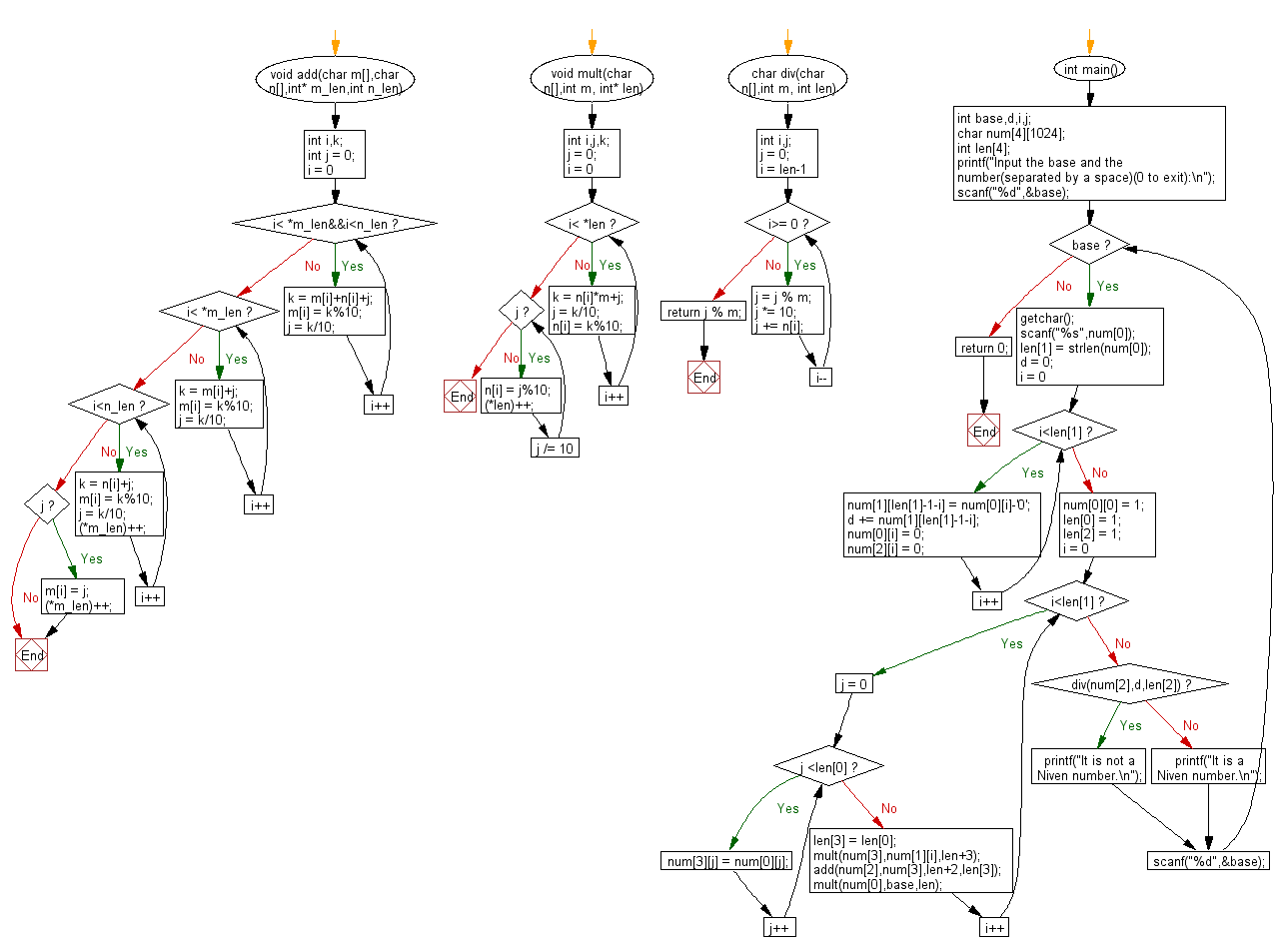﻿ C Program: Check a number with base b is Niven number# C Exercises: Check whether a given number with base b is a Niven number or not

## C Numbers: Exercise-38 with Solution

Write a C programming to check whether a given number with base b (2 <= b<= 10) is a Niven number or not.
From Wikipedia,
In recreational mathematics, a harshad number (or Niven number) in a given number base, is an integer that is divisible by the sum of its digits when written in that base. Harshad numbers in base n are also known as n-harshad (or n-Niven) numbers. Harshad numbers were defined by D. R. Kaprekar, a mathematician from India. The word "harshad" comes from the Sanskrit harṣa (joy) + da (give), meaning joy-giver. The term “Niven number” arose from a paper delivered by Ivan M. Niven at a conference on number theory in 1977. All integers between zero and n are n-harshad numbers.
The number 18 is a harshad number in base 10, because the sum of the digits 1 and 8 is 9 (1 + 8 = 9), and 18 is divisible by 9 (since 18/9 = 2, and 2 is a whole number).

Test Data
Input: base 10: Number 3
Output: 3 is a Niven Number
Input: base 10: Number 18
Output: 18 is a Niven Number
Input: base 10: Number 15
Output: 15 is not a Niven Number

Sample Solution:

C Code:

``````#include <stdio.h>
#include <string.h>

void add(char m[],char n[],int* m_len,int n_len)
{
int i,k;
int j = 0;
for(i = 0; i< *m_len&&i<n_len; i++)
{
k = m[i]+n[i]+j;
m[i] = k%10;
j = k/10;
}
for(;i< *m_len; i++)
{
k = m[i]+j;
m[i] = k%10;
j = k/10;
}
for(;i<n_len; i++)
{
k = n[i]+j;
m[i] = k%10;
j = k/10;
(*m_len)++;
}
if(j)
{
m[i] = j;
(*m_len)++;
}
}
void mult(char n[],int m, int* len)
{
int i,j,k;
j = 0;
for(i = 0; i< *len; i++)
{
k = n[i]*m+j;
j = k/10;
n[i] = k%10;
}
for(;j;j /= 10)
{
n[i] = j%10;
(*len)++;
}
}
char div(char n[],int m, int len)
{
int i,j;
j = 0;
for(i = len-1; i>= 0; i--)
{
j = j % m;
j *= 10;
j += n[i];
}
return j % m;
}
int main()
{
int base,d,i,j;
char num;
int len;
printf("Input the base and the number(separated by a space)(0 to exit):\n");
scanf("%d",&base);
while(base)
{
getchar();
scanf("%s",num);
len = strlen(num);
d = 0;
for(i = 0; i<len; i++)
{
num[len-1-i] = num[i]-'0';
d += num[len-1-i];
num[i] = 0;
num[i] = 0;
}
num = 1;
len = 1;
len = 1;
for(i = 0; i<len; i++)
{
for(j = 0; j <len; j++)
{
num[j] = num[j];
}
len = len;
mult(num,num[i],len+3);
mult(num,base,len);
}
if(div(num,d,len))
{
printf("It is not a Niven number.\n");
}
else
printf("It is a Niven number.\n");
scanf("%d",&base);
}
return 0;
}
```
```

Sample Output:

``` Input the base and the number(separated by a space)(0 to exit): 10 3 0
It is a Niven number.
```

Flowchart:C Programming Code Editor:

What is the difficulty level of this exercise?

Test your Programming skills with w3resource's quiz.

﻿

## C Programming: Tips of the Day

Why do C and C++ compilers allow array lengths in function signatures when they're never enforced?

It is a quirk of the syntax for passing arrays to functions.

Actually it is not possible to pass an array in C. If you write syntax that looks like it should pass the array, what actually happens is that a pointer to the first element of the array is passed instead.

Since the pointer does not include any length information, the contents of your [] in the function formal parameter list are actually ignored.

Ref : https://bit.ly/3fhlvdH